Home MonkeyNotes Printable Notes Digital Library Study Guides Study Smart Parents Tips College Planning Test Prep Fun Zone Help / FAQ How to Cite New Title Request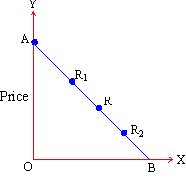Quantity demanded Figure 6 In the figure, AB is the demand curve and at any point on this, the elasticity of demand can be measured. At points R1, R and R2 the values of elasticity are: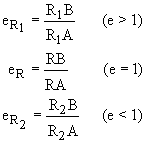At the mid point R on the demand curve, the value of elasticity is unit or equal to one. But above point R such as at R1, the value of elasticity is more than one and demand is highly elastic. On the other hand at a lower point such as R2 demand becomes inelastic as the value of elasticity is less than one. In general as we move in the direction of the Y axis, demand becomes more and more elastic. But as we move in the direction of the X axis, demand becomes less and less elastic. In other words at every higher price demand is relatively more elastic and at every lower price demand is relatively less elastic. This also explains that elasticity of demand differs not only from commodity to commodity but also for the same commodity at varying prices.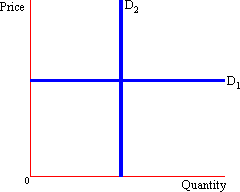b) Two extreme cases: Besides the three explained above, two more extreme values of price elasticity of demand can be included in the analysis. These are: (i) Perfectly Price Elastic: At this extreme, for any small decrease in price, the increase in the quantity demanded is infinitely large. In such a case, demanders demand all the can. Here the demand is said to be perfectly price elastic (e = that is infinity). This is represented graphically as a horizontal demand curve (D1 in the figure above). (ii) Perfectly Price Inelastic: At this extreme, for any change in price there is no change in the quantity demanded. Therefore the demand is completely unresponsive to any change in price. In this case the demand is said to be perfectly price inelastic (e = 0). This is represented graphically by a vertical demand curve (D2 in the figure above). ii) Elasticity of Supply: Like demand, elasticity of supply can also be classified into two major divisions: one the highly elastic, unitary elastic and highly inelastic type and two, the extreme cases of the perfectly elastic and the perfectly inelastic type. a) Highly elastic, unitary elastic and highly inelastic: Elasticity of supply can similarly be defined and computed at varying prices and quantities supplied. Elasticity of supply is the degree of responsiveness with which quantity supplied changes with a given change in the price. This can be expressed with a similar formula: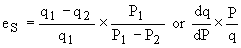An important difference between the price elasticity of demand and that of supply is that the latter is positive in value (as against the negative value in case of elasticity of demand). This is obvious from the fact that supply is a direct function of price: and both quantity and price change in the same direction. This will be clear from the following example. The values of q and P have been selected from the supply schedule given above.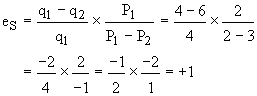The elasticity of supply also shows variations in its value for different commodities. Accordingly supply elasticity for different goods can be unit, (es = 1) more than one (es > 1) or less than one (es < 1). The goods can then be categorized as relatively elastic or inelastic in supply. Elasticity of supply is also of considerable practical importance in its policy applications. b) Two extreme cases: Besides the three explained above, two extreme values of price elasticity of supply can be included in the analysis: i) Perfectly Price Elastic: At this extreme for any small decrease in price, the quantity supplied is infinitely large. In such a case, suppliers supply all they can. Here the supply is said to be perfectly price elastic (e = that is infinity). This is represented graphically by a horizontal supply curve (S1 in the figure below). ii) Perfectly Price Inelastic: At this extreme for any change in price there is no change in the quantity supplied. Therefore the supply is completely indifferent to any change in price (e = 0). Here the supply is said to be perfectly price inelastic. This is represented graphically by a vertical supply curve (S2 in the figure below).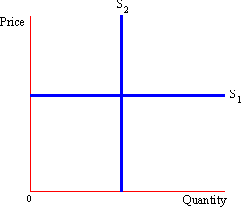Figure 8 Index 2. 1 Fundamental Concepts 2. 2 Demand Schedule, Function and Law 2. 3 Supply Schedule, Function and Law 2. 4 Elasticity of Demand and Supply 2. 5 The Concept of Equilibrium Chapter 3
 Search: All Products Books Popular Music Classical Music Video DVD Toys & Games Electronics Software Tools & Hardware Outdoor Living Kitchen & Housewares Camera & Photo Cell Phones Keywords: